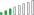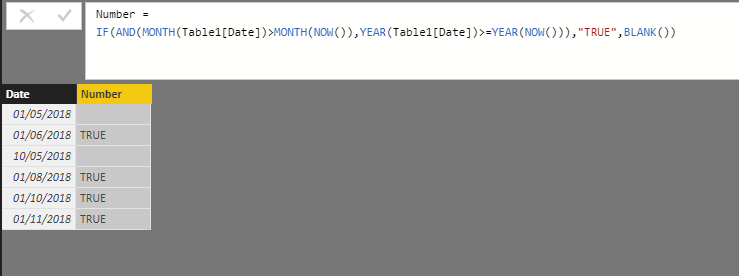cancel
Showing results for
Did you mean:Helper II

## Dax Formula Help

Hi there ;

İ just want to create a new formula but i can nat create because i am very new at DAX .

Coluld you pls help me about below issue , thanks for your supports.

Issue :

I have one column with dates as below.İ want to see that , if the date column's date is after this month ( the month that we are in)  , it will write to  near cell "true" in number column.İf not  the cell will be empty in new column.

İ want like that :1 ACCEPTED SOLUTIONResolver III

Hi,

Just add a calculated column and use that DAX formula :

Number =
IF(AND(MONTH(Table1[Date])>MONTH(NOW()),YEAR(Table1[Date])>=YEAR(NOW())),"TRUE",BLANK())2 REPLIES 2Resolver III

Hi,

Just add a calculated column and use that DAX formula :

Number =
IF(AND(MONTH(Table1[Date])>MONTH(NOW()),YEAR(Table1[Date])>=YEAR(NOW())),"TRUE",BLANK())Helper II

Hi drors ;

Thank you very much for support.